Practice Test: Civil Engineering (CE)- 2

# Practice Test: Civil Engineering (CE)- 2 - Civil Engineering (CE)

Test Description

## 65 Questions MCQ Test GATE Civil Engineering (CE) 2023 Mock Test Series - Practice Test: Civil Engineering (CE)- 2

Practice Test: Civil Engineering (CE)- 2 for Civil Engineering (CE) 2023 is part of GATE Civil Engineering (CE) 2023 Mock Test Series preparation. The Practice Test: Civil Engineering (CE)- 2 questions and answers have been prepared according to the Civil Engineering (CE) exam syllabus.The Practice Test: Civil Engineering (CE)- 2 MCQs are made for Civil Engineering (CE) 2023 Exam. Find important definitions, questions, notes, meanings, examples, exercises, MCQs and online tests for Practice Test: Civil Engineering (CE)- 2 below.
Solutions of Practice Test: Civil Engineering (CE)- 2 questions in English are available as part of our GATE Civil Engineering (CE) 2023 Mock Test Series for Civil Engineering (CE) & Practice Test: Civil Engineering (CE)- 2 solutions in Hindi for GATE Civil Engineering (CE) 2023 Mock Test Series course. Download more important topics, notes, lectures and mock test series for Civil Engineering (CE) Exam by signing up for free. Attempt Practice Test: Civil Engineering (CE)- 2 | 65 questions in 180 minutes | Mock test for Civil Engineering (CE) preparation | Free important questions MCQ to study GATE Civil Engineering (CE) 2023 Mock Test Series for Civil Engineering (CE) Exam | Download free PDF with solutions
 1 Crore+ students have signed up on EduRev. Have you?
Practice Test: Civil Engineering (CE)- 2 - Question 1

### Which of the following states the meaning of the idiom ‘Into the bargain’?

Detailed Solution for Practice Test: Civil Engineering (CE)- 2 - Question 1
‘Into the bargain’ has the meaning‘ in addition to what has already been mentioned or was expected’.
Practice Test: Civil Engineering (CE)- 2 - Question 2

### Direction: Find the correct relationship between the given quantities-Quantity I: Let E be the set of all integers with 6 in their unit place. The probability that a number chosen from the set {10, 11, 12, ........59} is a member of E is?Quantity II: A boy speaks truth in 70% of cases while another boy speaks truth in 65% cases. The probability that they will contradict each other on stating the same fact is?

Detailed Solution for Practice Test: Civil Engineering (CE)- 2 - Question 2
Quantity I: The sample space is n(s) = 50

The favourable outcomes are {16, 26, 36, 46, 56} i.e. 5

Probability = 5/50 = 0.1

Quantity II: Boy 1 speaks truth in 70% cases so 70/100 = 7/10 and

Boy 2: 65/100 = 13/20

Boy 2 does not speak truth in 35/100 cases i.e. 7/20

Probability = p (A')×p (B) +p (A)×p (B’)

[7×7/ (10×20)]+ [3×13/ (10×20)]

= 0.44

Practice Test: Civil Engineering (CE)- 2 - Question 3

### A square metallic sheet of an area of 1296 square cm is used for making the toys. For taking the metallic sheet from that square – four equal sized circular plates have been cut –off. If a fencing with the Silver thread is required to make the base of toys, how many rolls of the thread is required, if a roll contains 30 cm of the thread only?

Detailed Solution for Practice Test: Civil Engineering (CE)- 2 - Question 3
Side × Side = 1296

⇒ Side = 36

Radius of each circular plate = ¼ of 36 = 9 cm

Circumference of each plate = 2 π 9 = 56.57 cm

Total Circumference = 4 ×56.57 = 226.29 cm

No. of rolls = 226.29/30 = 7.54 = 8 rolls

Practice Test: Civil Engineering (CE)- 2 - Question 4

In the following question, some part of the sentence may have errors. Find out which part of the sentence has an error and select the appropriate option. If a sentence is free from error, select ‘No Error’.

I suppose no place is more (1)/ better than (2)/ home on Christmas. (3)/ No error (4)

Detailed Solution for Practice Test: Civil Engineering (CE)- 2 - Question 4
The error is in part (1) of the sentence. The use of "better" signifies that the sentence is of comparative degree. So, the use of "more" is incorrect here as it is redundant to be used with "better". So, remove "more".
Practice Test: Civil Engineering (CE)- 2 - Question 5

Direction: Read the pie chart given below and answer the following questions-

The pie chart given shows the population of India in the 7 states considering there are no other states apart from these.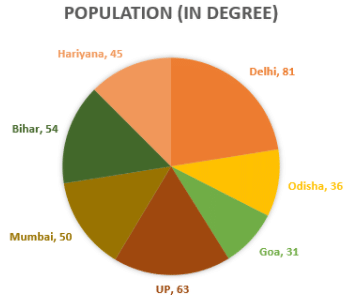Q. How much percent more is the population of UP than that of Odisha?

Detailed Solution for Practice Test: Civil Engineering (CE)- 2 - Question 5
Population of UP more than Odisha = 63⁰- 36⁰ = 27⁰,

Now for percentage we have to calculate how much more the population is in UP with respect to Odisha. So,

% more= (27⁰×100)/ 36⁰

= 75%

Practice Test: Civil Engineering (CE)- 2 - Question 6

In a class test, A scored 51 marks. The test had 4 sections. In the second section, A scored 7 marks more than in the first section, and in the third section, A scored 7 marks more than in the second section. If A did not score at all in the fourth section, how much did A score in the second section?

Detailed Solution for Practice Test: Civil Engineering (CE)- 2 - Question 6

If A scored x marks in section 2, he scored (x-7) marks in section 1 and (x+7) marks in section 3.

∴ (x-7) + x + (x+7) + 0 = 51

∴ 3x = 51

∴ x = 17

Hence, option is d.

Practice Test: Civil Engineering (CE)- 2 - Question 7

Direction: Study the information carefully and answer the questions given below.

P is standing north of Q at a distance of 6m. R is standing toward east of P at a distance of 6m. R is to the north of S and the distance between them is 6m. P is facing north direction, R is facing east, S is facing North and Q is facing towards west direction. All of them walk straight for 3m.

Q. What is the distance between the final position of P and Q?

Detailed Solution for Practice Test: Civil Engineering (CE)- 2 - Question 7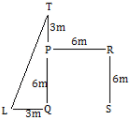Let T be the final position of P and L be the final position of Q.

Now LT = √(32 + 92) = √(9 + 81) = √ 90 = 3√10m

Practice Test: Civil Engineering (CE)- 2 - Question 8

In the following question, a statement has been given followed by two arguments numbered I and II. Based on the argument, you have to decide which of the following arguments is strong and which is a weak argument.

Statement: Shiva trilogy written by Amish Tripathi is the best fiction book.

Arguments

I. Yes, None other fiction books have three parts.

II. No, the best fiction book is subjective and it always differs from person to person.

Detailed Solution for Practice Test: Civil Engineering (CE)- 2 - Question 8

Best fiction book or novel is a subjective thing and differs from person to person. Hence, the second argument is strong.

Practice Test: Civil Engineering (CE)- 2 - Question 9

In the following question, some parts of the sentence may have errors. Find out which part of the sentence has an error and select the appropriate option. If a sentence is free from error, select ‘No Error’.

If this be overlooked, a wrong (1)/ impression may be derived as (2)/ to the most absolute amplitudes of the changes. (3)/ No error (4)

Detailed Solution for Practice Test: Civil Engineering (CE)- 2 - Question 9

The degree adjective ‘most’ can’t be used with absolute as absolute itself means complete or total. So remove most to make the sentence correct. Hence option C would be the correct answer.

Practice Test: Civil Engineering (CE)- 2 - Question 10

Direction: Following bar graphs show time taken by different pipes to fill that particular percentage of tank which is described in tabular data. For Ex- Pipe 1 fills the 10 % of the tank in 6 mins.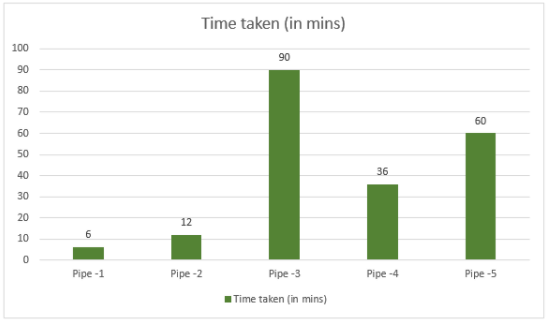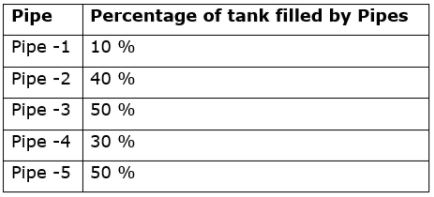A Tank has three pipes; two of them are used to fil the tank while another one used to empty the tank namely: pipe 3, pipe 5 to fill the tank and a new third pipe for making the tank empty. When all three pipes are open, 7/18th part of the tank is filled in 1 hours. What is the ratio of the efficiency of Pipe-3: Pipe 5: all three pipes working together.

Detailed Solution for Practice Test: Civil Engineering (CE)- 2 - Question 10

Part of work in one hour =

(P3+P5+Third pipE. X 1 = 7/18th part of work

⇒ 7/18th part of work = 1 hr

⇒ Total work done = 18/7 hr by all three pipes

Pipe 3 does 50% of work in 90 mins

100 % of work = 90/50 * 100 = 180 mins = 3 hr

Pipe 5 does 50% of work in 60 mins

100 % of work = 60/50 * 100 = 120 mins = 2 hr

Time Tw Efficiency

Pipe -3 = 3 hr Total work

Pipe-5 = 2 hr Total work

All three = 18/7 hr Total work

Hence efficiency ratio: 6: 9: 7.

Practice Test: Civil Engineering (CE)- 2 - Question 11

For a complex number z, find the value of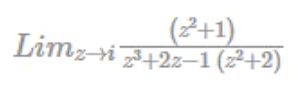Detailed Solution for Practice Test: Civil Engineering (CE)- 2 - Question 11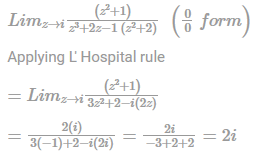Practice Test: Civil Engineering (CE)- 2 - Question 12

The velocity potential for a two-dimensional flow is given by ϕ = x2-y2+3xy. The flow rate between the streamlines passing through points (1, 2) and (2, 3) is

Detailed Solution for Practice Test: Civil Engineering (CE)- 2 - Question 12

ϕ = x2 - y2 + 3xy

⇒ ∂ϕ/∂x = u = 2x + 3y = ∂ψ/∂y

⇒ ψ = 2xy + 3/2y2 + f(x).... (1)

and,

- ∂ϕ/∂y = V = -2y + 3X = -∂ψ/∂x ...(ii)

And from (1)

-∂ψ/∂x = 2y-f'(x) = -2y+3x

⇒ f'(x) = -3x

⇒ f'(x) = -3/2x2

The required stream function is

ψ = 2xy- 3/2 (x2-y2)

At point (2,3);

ψ2 = 2×2×3-32(22-32)

= 12 - 3/2(-5) = 12+7.5 = 19.5 units

Flow rate between the streamlines passing through (1, 2) and (2, 3)

∆ψ = ψ2 - ψ1

= 19.5-8.5 = 11 units.

Practice Test: Civil Engineering (CE)- 2 - Question 13

A velocity field is given as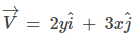where x and y are in metres. The acceleration(magnitude) of a fluid particle at (x, y) = (1, 1) is ___.

Detailed Solution for Practice Test: Civil Engineering (CE)- 2 - Question 13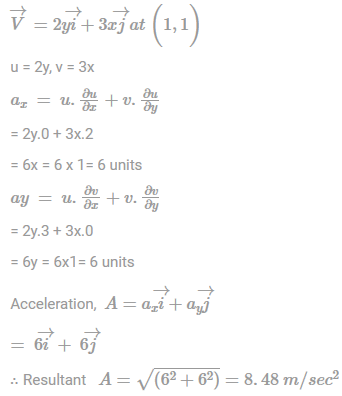Practice Test: Civil Engineering (CE)- 2 - Question 14

The matrix A is 3 × 3 matrix, if the matrix is a singular matrix then which of the following statement is true;

Detailed Solution for Practice Test: Civil Engineering (CE)- 2 - Question 14
For singular matrix, the determinant of matrix is zero

Also product of eigen values = det(matrix)

∴ product of eigen values will be zero, if at least one eigen value is zero.

Practice Test: Civil Engineering (CE)- 2 - Question 15

Consider the following function.

F(x) = (1/3)x3 + (1/2)x2 - 6x + 1000

Find the value at which f has a relative minimum.

Detailed Solution for Practice Test: Civil Engineering (CE)- 2 - Question 15
f(x) = (1/3)x3 + (1/2)x2 - 6x+1000;

f'(x) = x2+ x - 6 ⇒ x = -3.2

f''(x) = 2x+1

f''(-3) = 2(-2)+1 = -5<0

f''(2) = 2×2+1 = 5>0

⇒ f has relative maximum at x = -3

⇒ f has relative minimum at x = 2

Practice Test: Civil Engineering (CE)- 2 - Question 16

Over 1500 students appeared for the exam. The entrance exam has 100 multiple choice questions, each carrying 1 mark. Each question has 4 options. Due to negative marking, every incorrect choice gets –0.33 marks. Suppose 800 students choose all their answers randomly keeping uniform probability. The expected marks for 100 questions is

Detailed Solution for Practice Test: Civil Engineering (CE)- 2 - Question 16
The probability distribution for X is given below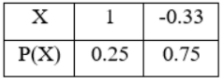Expected marks for one question, E(X) = ∑ X P (X)

= 1(0.25) + (-0.33)(0.75)

E(X) = 0.0025

Expected marks for 100 questions = 100 × 0.0025 = 0.25

Practice Test: Civil Engineering (CE)- 2 - Question 17

Given a vector field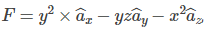, the one integral ∫F.dl evaluated along a segment on the x-axis from x = 1 to x = 2 is

Detailed Solution for Practice Test: Civil Engineering (CE)- 2 - Question 17
To find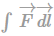along a segment on the x-axis from x = 1 to x = 2.

i.e., y = 0, z = 0, dy = 0 and dz = 0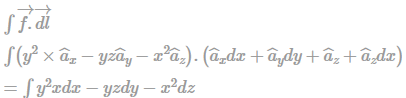Putting,y = 0,z = 0, dy = 0 and dz = 0

We get,

∫F→.d→l = 0.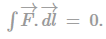Practice Test: Civil Engineering (CE)- 2 - Question 18

Match the following:

Group–I

P Rainfall intensity

Q Rainfall excess

R Rainfall averaging

S Mass curve

Group–II

1. Isohyets

2. Cumulative rainfall

3. Hyetograph

4. Direct runoff hydrograph

Detailed Solution for Practice Test: Civil Engineering (CE)- 2 - Question 18
A. Rainfall intensity versus time provided by Hyetograph.

B. Rainfall excess – Provides the direct run-off hydrograph over the infiltration index.

C. Rainfall average – Isohyets provides the time on the map having equal rainfall depth and used to measure the average rainfall over an catchment.

D. Mass curve – Anything which is cumulative is known as mass curve and cumulative rainfall is denoted by mass curve.

Practice Test: Civil Engineering (CE)- 2 - Question 19

An L = beam is shown in the figure below and is subjected to a factored shear force of 200 kN.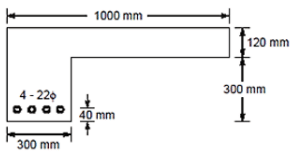Design shear strength of concrete, τc, for M20 grade of concrete is as given below in the table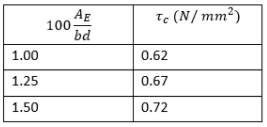Use Fe415 steel

The design shear stress for vertical stirrups is ____ N/mm2.

Detailed Solution for Practice Test: Civil Engineering (CE)- 2 - Question 19
Given data, Width of web = 300 mm

Effective depth = 120 + 300 – 40 = 380 mm

Percentage of tension steel,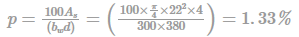From table of design shear strength,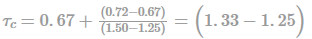= 0.69 N / mm2

Nominal shear stress,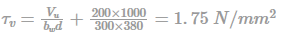Design shear stress,

τus = 1.75-0.69 = 1.06N/mm2.

Practice Test: Civil Engineering (CE)- 2 - Question 20

A layer of a beam at a distance y from the neutral layer is considered. If the beam is subjected to simple bending, and the radius of neutral is constant. Which of the following is correct?

Detailed Solution for Practice Test: Civil Engineering (CE)- 2 - Question 20
Strain in a layer = Increase in length / original length = y/R

Since R = radius of neutral axis, is constant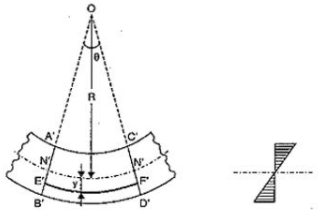Bending strain proportional to its distance to the neutral axis is assumption and it is applicable to thin beams, i.e. where the strain from shear can be ignored.

Practice Test: Civil Engineering (CE)- 2 - Question 21

Which assumptions are incorrect in column theory?

Detailed Solution for Practice Test: Civil Engineering (CE)- 2 - Question 21
Column buckling consists of finding maximum load on columns which support before it collapses. Its key feature is the comparison of internal bending moment arising from the internal stress distribution to the external bending moment resulting from the load applied to the column. The length of the column is very large as compared to the cross-sectional dimensions of the column.
Practice Test: Civil Engineering (CE)- 2 - Question 22

The value of P that results in the collapse of the beam shown in the figure below and having plastic moment capacity of MP is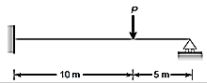Detailed Solution for Practice Test: Civil Engineering (CE)- 2 - Question 22
Number of possible hinges, n = 2

Statical indeterminacy, r = 1

Number of independent mechanisms,

I = n – r

= 2 – 1 = 1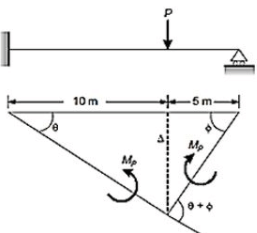∆ = 10 θ = 5ϕ

By principle of virtual work, we get

∆ × P - Mpθ - Mp(θ + ϕ) = 0

⇒ P × 10θ = 2Mpθ + Mpθ

⇒ P × 10θ = 2Mp + Mp × 10θ/5

⇒ 10P = 2Mp + 2Mp

∴ P = 0.42 Mp

Practice Test: Civil Engineering (CE)- 2 - Question 23

In order to determine the relative density of a sand sample, its natural moisture content and bulk density were determined in the field and were found to be 7% and 1.61 g/cc respectively. Sample of this soil was then commented in a Proctor’s mould of 943.89 cc capacity at the loosest and the densest states. The following data were obtained:

1) Weight of empty mould = 2100 g

2) Weight of mould + soil in the loosest state = 3363.6 g

3) Weight of mould + soil in the densest state = 3857.4 g

4) Moisture content of the sample used in tests = 11%

Q. The relative density of sand is ______%.

Detailed Solution for Practice Test: Civil Engineering (CE)- 2 - Question 23
In the loosest state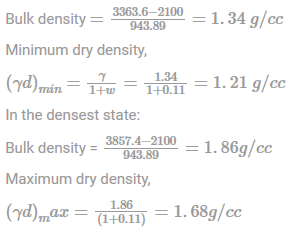In-situ density of the soil = 1.61 g/cc

W = 7%

∴ In-situ dry density,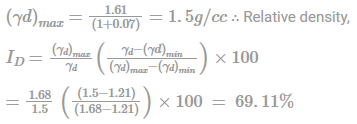Practice Test: Civil Engineering (CE)- 2 - Question 24

A city has a population of 10, 00,000. Average water consumption of the people is 270 lpcd, then the maximum hourly demand in MLD is

Detailed Solution for Practice Test: Civil Engineering (CE)- 2 - Question 24
Average daily demand (Qavg)=Population × Per capita water demand

= 10,00,000 × 270 = 270 MLD = 270/24= 11.25 ML per hour

(Qmax)hourly= Qavg × 2.7=270 × 2.7=729 MLD

Maximum hourly demand = 2.7 x Average Hourly Demand

= 2.7 x 11.25 ML per hour = 30.375 ML per hour= 30.375 x 24 MLD

= 729 MLD

Practice Test: Civil Engineering (CE)- 2 - Question 25

If the sensitivity of the bubble tube of a level is 30" of arc per division, then the distance of a point at which the combined curvature and refraction corrections become numerically equal to the error induced by dishevelment of one division of the level tube (in m) is

Detailed Solution for Practice Test: Civil Engineering (CE)- 2 - Question 25
Sensitivity, ϕ = (s × 206265)/(L×n);

Where s, n and L combined curvature and refraction correction, no. of division of the level tube and distance of point under observation respectively.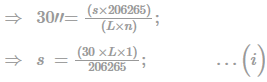Correction due to combined curvature and refraction

C = - 0.0673 L2 , where L is in km …(ii)

From equation (i) and (ii),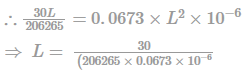∴ L = 2161.13 m

Practice Test: Civil Engineering (CE)- 2 - Question 26

On a plate u = 1/3 y - y2/6, where u is in m/sec & y is in m & μ of fluid = 0.5 Ns/m2. Shear stress at 0.4 m above plate and 0.4 m above plate are in ratio

Detailed Solution for Practice Test: Civil Engineering (CE)- 2 - Question 26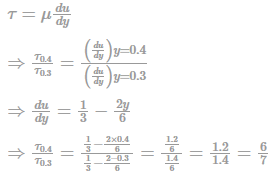*Answer can only contain numeric values
Practice Test: Civil Engineering (CE)- 2 - Question 27

The data given below pertain to the design of a flexible pavement.

Initial traffic = 1350 cvpd

Traffic growth rate = 7% per annum

Design life = 15 years

Vehicle damage factor = 2.50

Distribution factor = 1.05

The design traffic in terms of Million standard axles (MSA) to be catered would be.

Detailed Solution for Practice Test: Civil Engineering (CE)- 2 - Question 27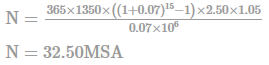Practice Test: Civil Engineering (CE)- 2 - Question 28

What will be the population equivalent for an industrial sewage daily disposal of 50,000 litres with suspended solid content of 220 mg/lit, considering the per capita contribution as 69 gm.

Detailed Solution for Practice Test: Civil Engineering (CE)- 2 - Question 28
Total solids produced by the Industry/day = (50000 x 220)/1000 =11,000 gm

Population equivalent = 11,000/69

= 159.

Practice Test: Civil Engineering (CE)- 2 - Question 29

A centrifugal pump will start delivering the liquid only when

Detailed Solution for Practice Test: Civil Engineering (CE)- 2 - Question 29
A centrifugal pump will start delivering the liquid only when the head developed tends to exceed the manometric head.
*Answer can only contain numeric values
Practice Test: Civil Engineering (CE)- 2 - Question 30

On an urban road, the free mean speed was measured as 70 kmph and the average spacing between the vehicles under jam condition as 7.0 m. The speed-flow -density equation is given by

U = Usf[1 − k/kj] and q = Uk

Where U = space-mean speed (kmph); Usf = free mean speed (kmph); k = density (veh/km); kj = jam density (veh/km); q = flow (veh/hr). The maximum flow (veh/hr) per lane for this condition is equal to

Detailed Solution for Practice Test: Civil Engineering (CE)- 2 - Question 30
kj = 1000s = 1000/7 = 142.86veh/km

qmax = vsfkj/4 = 70 × 142.864 = 2500veh/hr

Practice Test: Civil Engineering (CE)- 2 - Question 31

For flow of incompressible, viscous flow two configurations are shown. The inlet velocity for the diverging nozzle (Fig (i)) and free-stream velocity for flow past the bluff body (Fig(ii)) is constant and flow is laminar. The relation regarding velocity gradients at point A and B is (. Points A and B are separation points and y is the direction normal to the surface at the point of separation)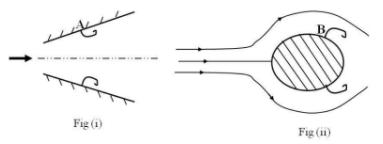Detailed Solution for Practice Test: Civil Engineering (CE)- 2 - Question 31
Points A and B are points where flow separation takes place. At both points Points ∂u/∂y = 0. Hence (∂u/∂y)A = (∂u/∂y)B = 0
Practice Test: Civil Engineering (CE)- 2 - Question 32

The assumption used to derive the expression ∫dP/ρ + v2/2 + gy = constant , is

Detailed Solution for Practice Test: Civil Engineering (CE)- 2 - Question 32
∫dP/ρ + v2/2 + gy = constant

This expression is obtained by integration of Euler’s equation. The Euler equation is integrated along the streamline in a steady, frictionless flow.

Practice Test: Civil Engineering (CE)- 2 - Question 33

Find the depth of centre of pressure if a rectangular plate of 12 m x 6 m is in water and the surface of the plate is 2 m below the water surface. Width is 6 m.

Detailed Solution for Practice Test: Civil Engineering (CE)- 2 - Question 33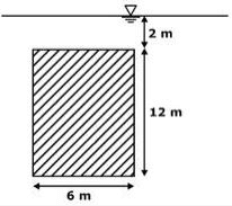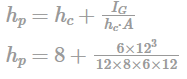= 9.5m

Practice Test: Civil Engineering (CE)- 2 - Question 34

Match List I and List II relating the forces and the related soil structure.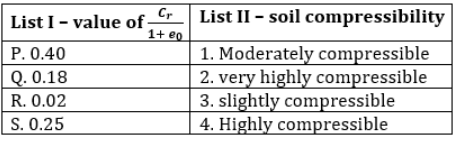Detailed Solution for Practice Test: Civil Engineering (CE)- 2 - Question 34
For over consolidated clay soil, the classification is done using the relation between the recompression index (Cr) and the void ratio (e0) of the soil sample.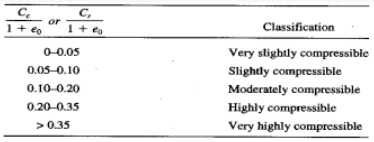Practice Test: Civil Engineering (CE)- 2 - Question 35

Determine the Hydraulic conductivity of a normally consolidated clay deposit of thickness 3m with one way drainage. The coefficient of volume compressibility is 5.79 x 10-4 m2/kN and coefficient of compressibility is 0.002 cm2/s.

Detailed Solution for Practice Test: Civil Engineering (CE)- 2 - Question 35
Coefficient of compressibility, Cv = k/mvxyw

K = coefficient of permeability

On substituting the values

Hydraulic conductivity, k = 0.113 x 10-6 cm/sec

Practice Test: Civil Engineering (CE)- 2 - Question 36

A ship has a metacentre height of 0.90 m and its period of rolling is 20 seconds. The relevant radius of gyration is nearly

Detailed Solution for Practice Test: Civil Engineering (CE)- 2 - Question 36
Time period,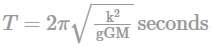GM = metacentric height = 0.90 m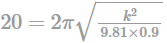k = 9.55 m

Practice Test: Civil Engineering (CE)- 2 - Question 37

What will be the bond stress developed for a steel bar of diameter 25 mm embedded 460 mm inside concrete. Pull applied on steel is 80 kN. Use M30 concrete and Fe 415 steel. Use the limit state method.

Detailed Solution for Practice Test: Civil Engineering (CE)- 2 - Question 37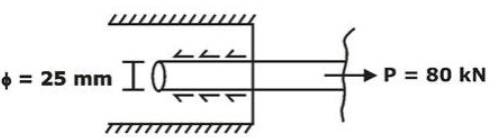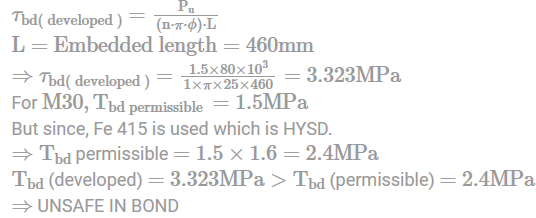*Answer can only contain numeric values
Practice Test: Civil Engineering (CE)- 2 - Question 38

If 5 unbiased dice are thrown simultaneously, the probability of obtaining exactly two sixes is

Detailed Solution for Practice Test: Civil Engineering (CE)- 2 - Question 38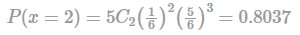*Answer can only contain numeric values
Practice Test: Civil Engineering (CE)- 2 - Question 39

In a certain animal husbandry weight of animals had a distribution as shown in table below: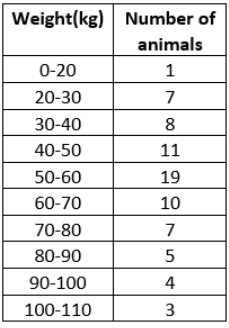Mean weight of animals in the husbandry is______.

Detailed Solution for Practice Test: Civil Engineering (CE)- 2 - Question 39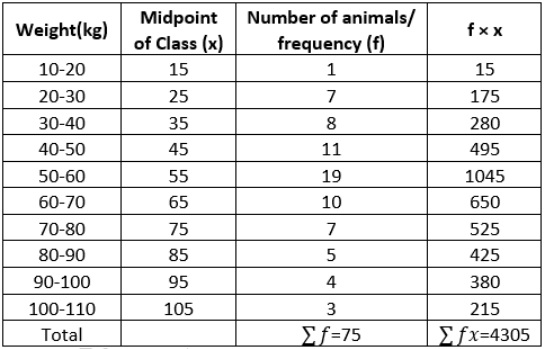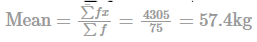Practice Test: Civil Engineering (CE)- 2 - Question 40

(D2−5D+6)y = ex cos⁡ x is a Partial differential equation whose solution is given by y =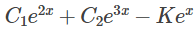(3sin⁡ 2x + cos ⁡2x)

The value of K is

Detailed Solution for Practice Test: Civil Engineering (CE)- 2 - Question 40

The objective here is to find the Particular integral as Complementary function is already given in the solution.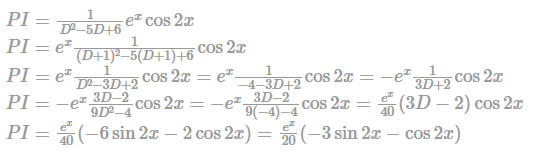*Answer can only contain numeric values
Practice Test: Civil Engineering (CE)- 2 - Question 41

Find the missing rainfall at station X. (in cm)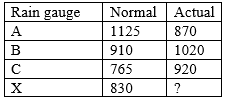Detailed Solution for Practice Test: Civil Engineering (CE)- 2 - Question 41
As the normal ppt of other station A, B and C are not within 10% of normal ppt of station X.830 × 1.1 = 913

Hence, we will use the normal ratio method.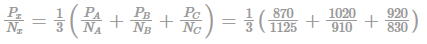Px = 830.732mm = 83.073cm

*Answer can only contain numeric values
Practice Test: Civil Engineering (CE)- 2 - Question 42

A jet of water comes from a 5 cm diameter nozzle, held vertically upwards, at a velocity of 20 m/sec. If air resistance consumes 10% of the initial energy of the jet, then it would reach at a height, above the nozzle of? Report the answer in cm.

Detailed Solution for Practice Test: Civil Engineering (CE)- 2 - Question 42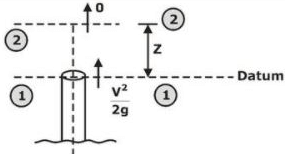Energy losses = 10% [Initial Energy]

= 0.1(V2/2g)

Since sections (1) – (1) & (2) – (2) both are subjected to the atmosphere, hence, the gauge pressure = 0.

Applying Bernoulli’s Equation between (1)-(1) & (2)-(2)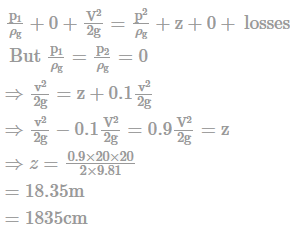*Answer can only contain numeric values
Practice Test: Civil Engineering (CE)- 2 - Question 43

The value of slope at A in the given beam is ______________× 10-3. (up to 1 decimal place)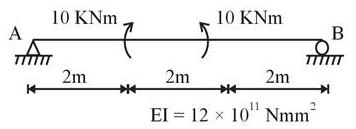Detailed Solution for Practice Test: Civil Engineering (CE)- 2 - Question 43
Using conjugate beam method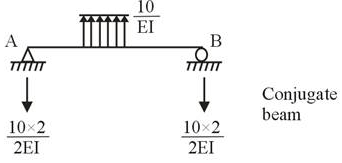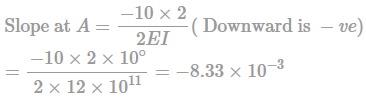Practice Test: Civil Engineering (CE)- 2 - Question 44

Determine the moment at fixed support for the beam shown in the figure.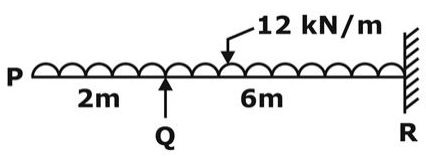Detailed Solution for Practice Test: Civil Engineering (CE)- 2 - Question 44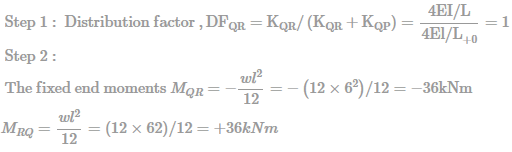Step 3: Moment distribution

Moment at Q considering the overhanging side, MQ = 12x2x(2/2) = 24kNm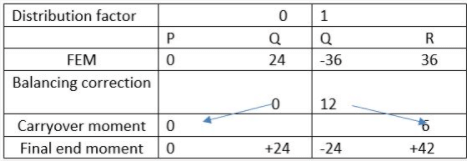Therefore the moment at the fixed end R = 42kNm

Practice Test: Civil Engineering (CE)- 2 - Question 45

If the lowest value of reinforcement is to be provided to a beam, then which of the following represents a permissible value according to 15:456−2000 in a beam of cross section 300 × 750mm

Detailed Solution for Practice Test: Civil Engineering (CE)- 2 - Question 45

Minimum value of area of steel in beam Ast/bd ≥ 0.85/fy

For Fe250 min area, Ast ≥ 0.85 × 300 × 750/250

Ast ≥ 765mm2

For Fe415 min area, Ast ≥ 0.85 × 300×750/415

Ast ≥ 460.84mm2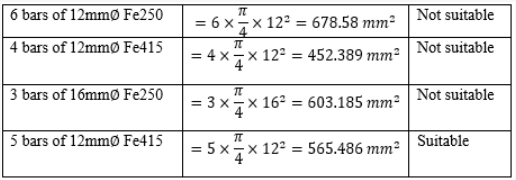*Answer can only contain numeric values
Practice Test: Civil Engineering (CE)- 2 - Question 46

For the rigid frame shown below, what is the moment reaction at A?

Support C is pinned.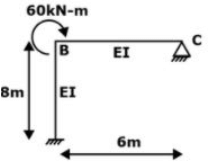Detailed Solution for Practice Test: Civil Engineering (CE)- 2 - Question 46
Relative stiffness of member BC

K1 = 3/4(1/6) = 1/8

Relative stiffness of member BA

K2 = 1/8

∑K = K1 + K2 = 1/8 + 1/8 = 1/4

Distribution factor (DF) for member BC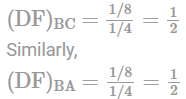i.e. MBA = (DF)BA × 60kN − m = 30kN −m

Thus, by carry over theorem

Moment at A = 1/2MBA = 30/2 = 15kN−m

Practice Test: Civil Engineering (CE)- 2 - Question 47

Choose the correct statements out of the followings

1) The shrinkage deflection depends upon the relative amount of Tension and compression reinforcement

2) In limit state method of design, the failure criterion for RCC beam and column is maximum principal stress theory

3) Failure by crushing concrete before yielding of steel is called secondary compression failure and failure by crushing of concrete after yielding is called primary compression failure.

4) Under reinforced section is deeper and can undergo larger deflection when compared to balanced and over-reinforced section.

Detailed Solution for Practice Test: Civil Engineering (CE)- 2 - Question 47

Correct statements are

1. The shrinkage deflection depends upon the relative amount of Tension and compression reinforcement

4. Under reinforced section is deeper and can undergo larger deflection when compared to balanced and over-reinforced section.

Practice Test: Civil Engineering (CE)- 2 - Question 48

From the beam shown below, the value of support moment M is ______ kN-m.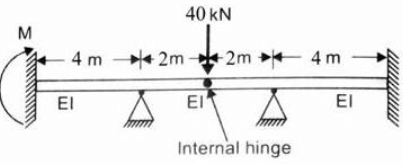Detailed Solution for Practice Test: Civil Engineering (CE)- 2 - Question 48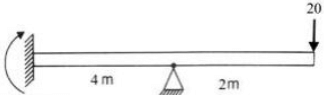M = 20KN - m

By carry over theorem,

M = 20 x 2/2 = 20 kN-m

Practice Test: Civil Engineering (CE)- 2 - Question 49

A thin walled cylindrical pressure vessel having a radius of 0.5 m and wall thickness of 25 mm is subjected to an internal pressure of 700 kPa. The hoop stress developed is

Detailed Solution for Practice Test: Civil Engineering (CE)- 2 - Question 49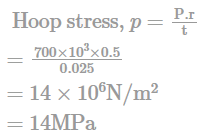Practice Test: Civil Engineering (CE)- 2 - Question 50

The possible location of shear centre of the channel section, shown below, is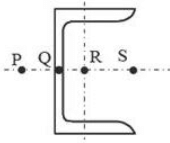Detailed Solution for Practice Test: Civil Engineering (CE)- 2 - Question 50

Shear centre is the point through which the internal flexural shear should pass. When external shear force due to loading passes through, at the same point, no torsion is caused.

For the section shown ‘P’ is the shear centre.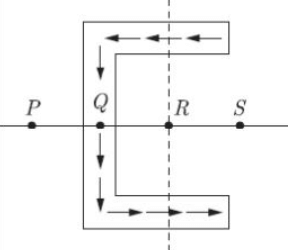Practice Test: Civil Engineering (CE)- 2 - Question 51

A foundation in a loose sand is 4 m wide, 6 m long and 1.5 m deep. The soil weighs 16 kN/m3 and has an effective angle of internal friction of 22.6°. Compute the safe bearing capacity of soil (in kN/m2), adopting a factor of safety of 2.

[for ϕ=22.6,

Nc = 21.55.Nq

= 10.16 and Nγ = 7.44]

Detailed Solution for Practice Test: Civil Engineering (CE)- 2 - Question 51

B = 4, L = 6m, Df = 1.5m

Since Df < b,="" it="" is="" a="" shallow="" footing.="" moreover,="" it="" is="" a="" rectangular="" footing="" with="" b/l="" />

Now, for rectangular footing, these factors must be corrected by multiplying with shape factors as: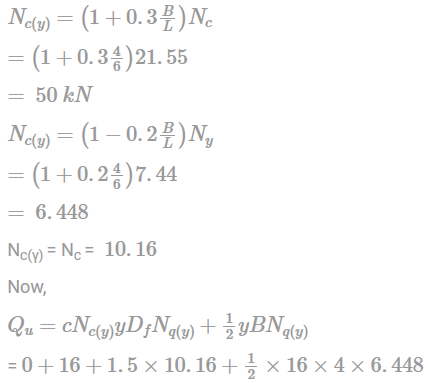(∵ c = 0 for loose and)

= 450.2 kN/m2

Safe bearing capacity,

qa = (Ultimate bearing capacity)/FOS

= 450.2/2

= 225.1 kN/m2

Practice Test: Civil Engineering (CE)- 2 - Question 52

The value of the integral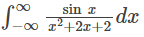Evaluated using contour integration and the residue theorem is;

Detailed Solution for Practice Test: Civil Engineering (CE)- 2 - Question 52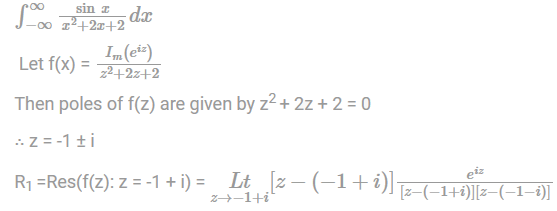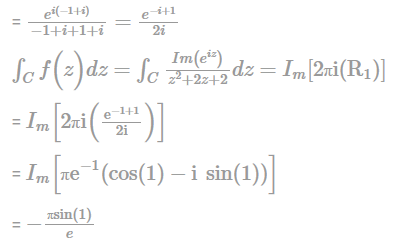Practice Test: Civil Engineering (CE)- 2 - Question 53

List-I below gives the possible types of failure for a finite soil slope and List-II gives the reasons for these different types of failure. Match the items in List-I with the items in List-II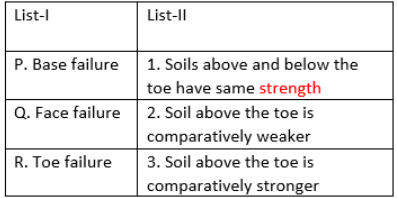Detailed Solution for Practice Test: Civil Engineering (CE)- 2 - Question 53

Base failure: Failure occurs along a surface that passes at some distance below the toe of the slope. That is possible when soil above the toe is comparatively stronger.

Face failure : Above the toe failure surface passes only possible when the soil above toe is comparatively weaker.

Toe failure: Passes through the toe of finite slope when above two failures like base or face failure are not possible, i.e., soil above and below the toe have the same strength.

Practice Test: Civil Engineering (CE)- 2 - Question 54

The magnetic bearing of a line (on full-circle mensuration basis) is 55 ° 30’ East. The true magnetic bearing of the line will be. (Given declination is 5 °30’ E)

Detailed Solution for Practice Test: Civil Engineering (CE)- 2 - Question 54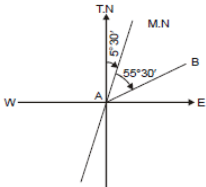T.B = 55 ° 30’ + 5 ° 30’

T.B = 61 °00'

Practice Test: Civil Engineering (CE)- 2 - Question 55

A 400 m long horizontal pipe is to deliver 1000 kg of oil (s = 0.9, ν = 0.0002 m2/s) per 60 seconds. If the head loss is not to exceed 800 cm of oil, the diameter of pipe is _____ m.

[Friction factor for laminar flow : f = 64/Rε]

Detailed Solution for Practice Test: Civil Engineering (CE)- 2 - Question 55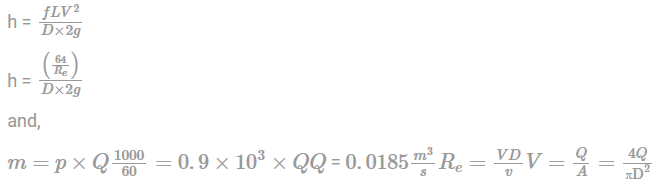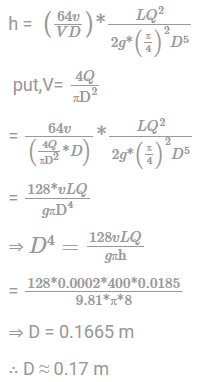Practice Test: Civil Engineering (CE)- 2 - Question 56

A rectangular 230 mm × 350 mm beam is (effective depth). The factored shear force acting at a section is 80 kN. If the permissible shear stress in concrete is 0·25 MPa, the design shear force is nearly

Detailed Solution for Practice Test: Civil Engineering (CE)- 2 - Question 56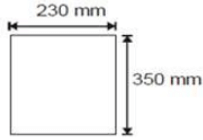Shear force resisted by concrete section only

(Fc)

= 230 × 350 × 0.25 × 10–3

= 20.125 kN

Design shear force (that will be resisted by shear stirrups)

Fd = factored shear force – Fc

Fd = 80 - 20.125

Fd = 59 - 875 kN

Fd = 60 kN

Practice Test: Civil Engineering (CE)- 2 - Question 57

A spillway is having 6 spans with clear width 10 m each. Thickness of each 90 ° cut water nose pier may be taken as 2.5 m. Take rounded abutment and effective height of water above spillway is 16.3 m. The effective length of spillway is _________ m.

Detailed Solution for Practice Test: Civil Engineering (CE)- 2 - Question 57

We know effective length, Le = L – 2 [Nkp + ka]He

For 90 ° cut water nose pier,

kp = 0.01, ka = 0.1

Number of piers, N = 5

So, Le = 60 – 2[5 x 0.01 + 0.1] x 16.3

Le = 55.1 m

Practice Test: Civil Engineering (CE)- 2 - Question 58

The value of the function is f(x)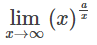Detailed Solution for Practice Test: Civil Engineering (CE)- 2 - Question 58

As we already have, y = abx = aexIn(b)

∴ xa/x e(a/x)In(x)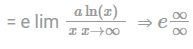Now,we can apply L' Hospital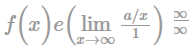= e1/∞ = 1

Practice Test: Civil Engineering (CE)- 2 - Question 59

For naturally occurring sand, the magnitude of maximum and the minimum void ratio varies widely. The main factor responsible for this wide variation is;

Detailed Solution for Practice Test: Civil Engineering (CE)- 2 - Question 59

For naturally occurring sands, the magnitude of emax and emin may vary widely mainly because of the Uniformity Coefficient (Cu) and the roundness of the particle.

Practice Test: Civil Engineering (CE)- 2 - Question 60

Water flows through a vertical contraction from a pipe of diameter d to another of diameter of d/2. Inlet velocity at inlet to contraction is

2 m/s and pressure 200 kN/m2. If height of contraction measures 2 m/s, the pressure at exit of contraction will be nearly to

Detailed Solution for Practice Test: Civil Engineering (CE)- 2 - Question 60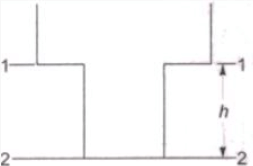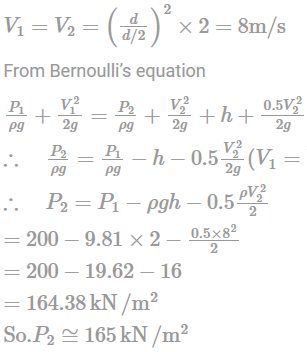Practice Test: Civil Engineering (CE)- 2 - Question 61

A hollow steel shaft 1.5 m long is to transmit a torque of 80 kN-m. What will be the inside diameter (in mm) of the shaft, if the angle of twist should not exceed 1 ° and the allowable shear stress is 90 MPa?

[Take G = 85 GPa]

Detailed Solution for Practice Test: Civil Engineering (CE)- 2 - Question 61

As we know that, θ = TL/GJ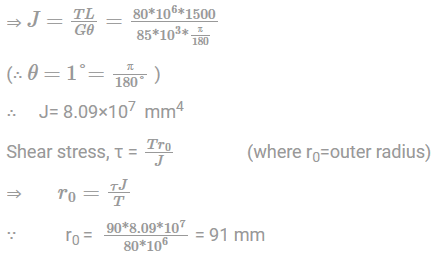Thus, outside diameter (D0) = 2 x r0 = 182 mm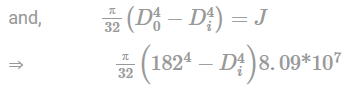∴ Inside diameter,Di = 128.56 mm

Practice Test: Civil Engineering (CE)- 2 - Question 62

The population of a city is 20000 with an average water demand of 150 lpcd. Calculate the area required {m2) of rapid sand filter system for the treatment of water. Daily 4% of filtered water is required for backwashing and it is being done for a duration of 30 minute. Given the Rate of filtration is 6000 Litre /hr/m2.

Detailed Solution for Practice Test: Civil Engineering (CE)- 2 - Question 62

Daily maximum water demand = 1.8 × 150 × 20000

= 5.4MLD

Total filtered water requirement =5.4/0.96

= 5.625MLD

30 minutes is lost every day in washing the filter, hence filtered water requirement per hour

= 5.625×106/23.5

= 239361.7l/hr

Area of filter required, A = 239361.7/6000

= 39.89m2

Practice Test: Civil Engineering (CE)- 2 - Question 63

Find the settlement of a footing of width 1.2m with the following plate lad test result for sandy soil. Settlement of plate is 12mm; width of plate is 400 mm.

Detailed Solution for Practice Test: Civil Engineering (CE)- 2 - Question 63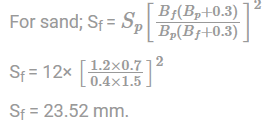Practice Test: Civil Engineering (CE)- 2 - Question 64

In a wide rectangular channel, the normal depth is increased by 20%. This would mean an increase in the discharge of the channel nearly by;

Detailed Solution for Practice Test: Civil Engineering (CE)- 2 - Question 64

For wide rectangular channel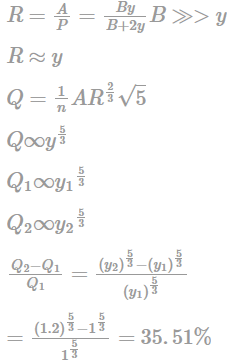Practice Test: Civil Engineering (CE)- 2 - Question 65

Consider the following linear system.

x + 2y - 3z = a

2x + 3y + 3z = b

5x + 9y - 6z = c

This system is consistent if a,b and c satisfy the equation

Detailed Solution for Practice Test: Civil Engineering (CE)- 2 - Question 65

(AB) = (1 2 -3 a 2 3 3 b 5 9 - 6 c)

(R2 - 2R1) ; (R3-5R1)

≈ (1 2 - 3 a 0 -1 9 b - 2a 0 -1 9 c - 5a)

(R3 - R2)

≈ (1 2 - 3 a 0 - 1 9 b - 2a 0 0 0 (c - b - 3a))

(c - b - 3a)

3a + b - c = 0

## GATE Civil Engineering (CE) 2023 Mock Test Series

27 docs|296 tests
Information about Practice Test: Civil Engineering (CE)- 2 Page
In this test you can find the Exam questions for Practice Test: Civil Engineering (CE)- 2 solved & explained in the simplest way possible. Besides giving Questions and answers for Practice Test: Civil Engineering (CE)- 2, EduRev gives you an ample number of Online tests for practice

## GATE Civil Engineering (CE) 2023 Mock Test Series

27 docs|296 tests(Scan QR code)